SPSS TUTORIALS BASICS ANOVA REGRESSION FACTOR CORRELATION

# Creating Boxplots in SPSS – Quick Guide

There's 3 ways to create boxplots in SPSS:

• GraphsLegacy DialogsBoxplot
• AnalyzeDescriptive StatisticsExplore
• GraphsChart Builder

The first approach is the simplest but it also has fewer options than the others. This tutorial walks you through all 3 approaches while creating different types of boxplots.

## Example Data

All examples in this tutorial use driving-test.sav, partly shown below.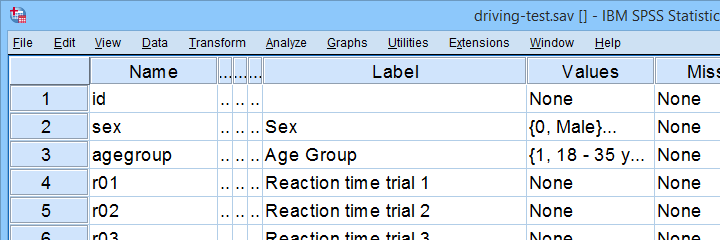Our data file contains a sample of N = 238 people who were examined in a driving simulator. Participants were presented with 5 dangerous situations to which they had to respond as fast as possible. The data hold their reaction times and some other variables.

## Boxplot for 1 Variable - 1 Group of Cases

We'll first run a boxplot for the reaction times on trial 1 for all cases. One option is GraphsLegacy DialogsBoxplot which opens the dialogs shown below.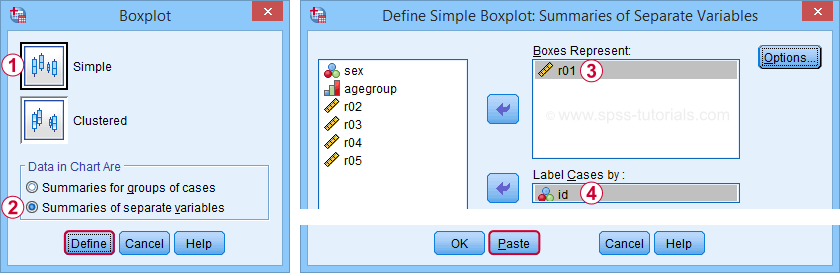Completing these steps results in the syntax below.

*Boxplot for r01 (all cases).

EXAMINE VARIABLES=r01
/COMPARE VARIABLE
/PLOT=BOXPLOT
/STATISTICS=NONE
/NOTOTAL
/ID=id
/MISSING=LISTWISE.

## Result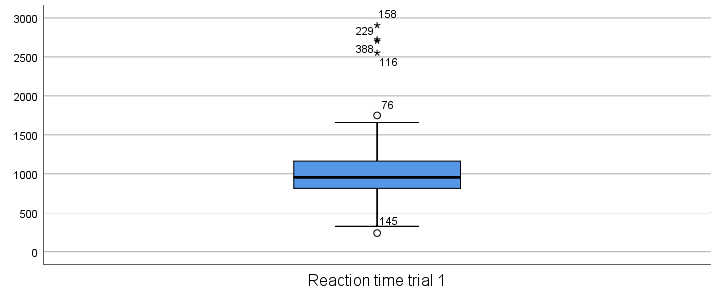Our boxplot shows some potential outliers as well as extreme values. Interpreting these -and all other boxplot elements- is discussed in Boxplots - Beginners Tutorial. Also note that our boxplot doesn't have a title yet. Options for adding it are discussed in Tip 3 - Adding Titles to Boxplots.

## Boxplot for Multiple Variables - 1 Group of Cases

We'll now create a single boxplot for our 5 reaction time variables for all participants. We navigate to AnalyzeDescriptive StatisticsExplore and fill out the dialogs as shown below.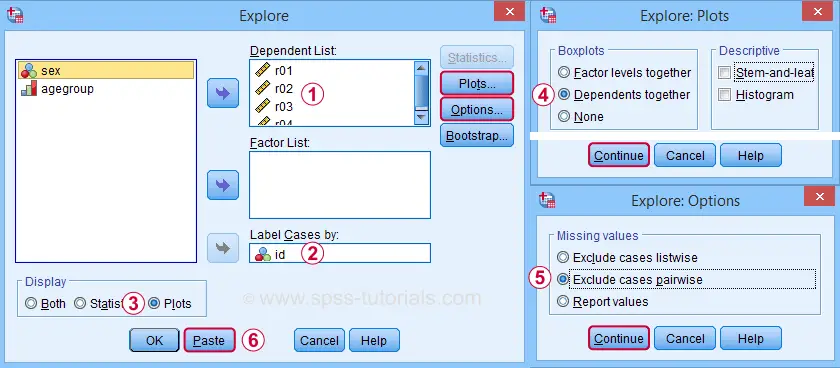“Dependents together” means that all dependent variables are shown together in each boxplot. If you enter a factor -say, sex- you'll get a separate boxplot for each factor level -female and male respondents. “Factor levels together” creates a separate boxplot for each dependent variable, showing all factor levels together in each boxplot.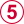“Exclude cases pairwise” means that the results for each variable are based on all cases that don't have a missing value for that variable. “Exclude cases listwise” uses only cases without any missing values on all variables.

A minor note here is that many SPSS users select “Normality plots and tests” in this dialog for running a

Anyway. Completing these steps results in the syntax below. Let's run it.

*Boxplot for comparing 5 variables on 1 group of cases.

EXAMINE VARIABLES=r01 r02 r03 r04 r05
/COMPARE VARIABLE
/PLOT=BOXPLOT
/STATISTICS=NONE
/NOTOTAL
/ID=id
/MISSING=PAIRWISE /* IMPORTANT! */.

## Result

Now, before inspecting our boxplot, take a close look at the Case Processing Summary table first.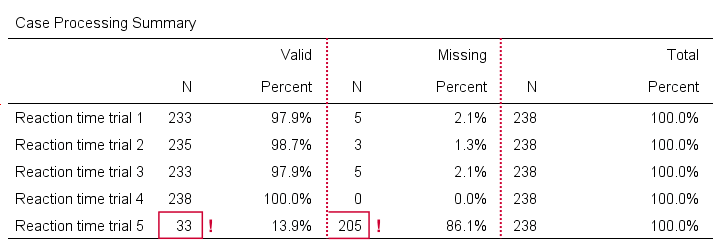The first columns tells how many cases were used for each variable. Note that trial 5 has N = 205 or 86.1% missing values. Remember that “Exclude cases listwise” was the default in the Explore dialog. If we hadn't changed that, then none of our variables would have used more than N = 33 cases. The actual boxplot, however, wouldn't show anything wrong. This really is a major pitfall. Please avoid it.

Anyway, the figure below shows our actual boxplot.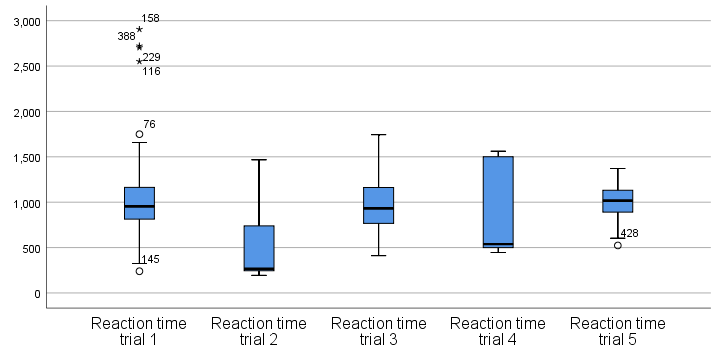Note that we already saw the first boxplot bar in our previous example. Second, trials 2 and 4 seem strongly positively skewed. Both variables look odd. We'd better inspect their histograms to see what's really going on.

## Boxplot for 1 Variable - Multiple Groups of Cases

We'll now run a boxplot for trial 3 for age groups separately. We first navigate to GraphsChart Builder and fill out the dialogs as shown below.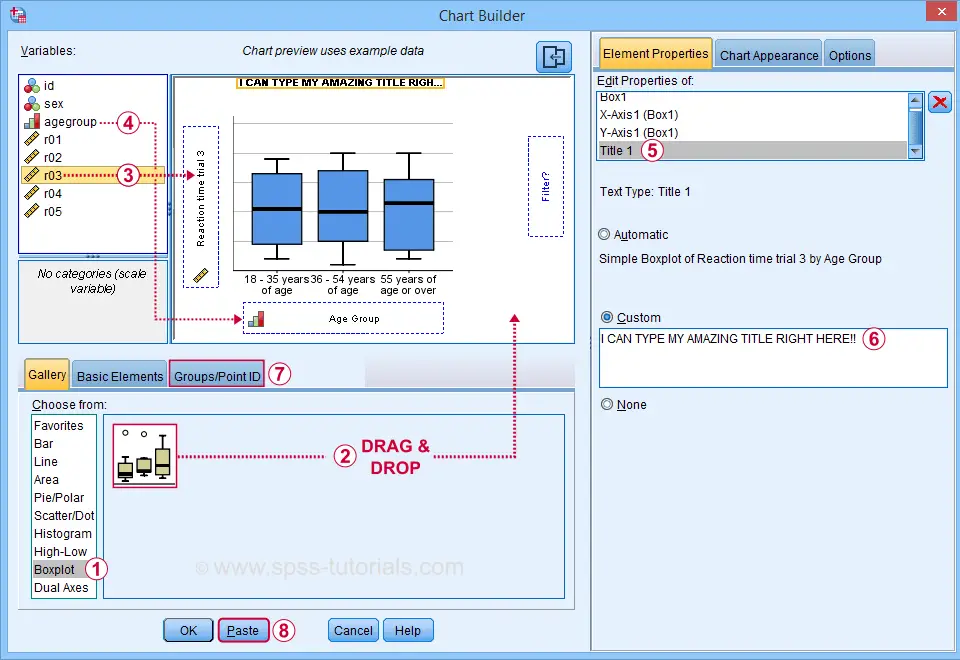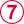Select “Point ID Label” in this tab and then drag & drop r03 into the ID box on the canvas. Doing so will show actual outlier values in the final boxplot.

Completing these steps results in the syntax below.

*Boxplot for comparing 3 age groups on 1 variable.

GGRAPH
/GRAPHDATASET NAME="graphdataset" VARIABLES=agegroup r03 MISSING=LISTWISE REPORTMISSING=NO
/GRAPHSPEC SOURCE=INLINE.
BEGIN GPL
SOURCE: s=userSource(id("graphdataset"))
DATA: agegroup=col(source(s), name("agegroup"), unit.category())
DATA: r03=col(source(s), name("r03"))
GUIDE: axis(dim(1), label("Age Group"))
GUIDE: axis(dim(2), label("Reaction time trial 3"))
GUIDE: text.title(label("I CAN TYPE MY AMAZING TITLE RIGHT HERE!"))
SCALE: cat(dim(1), include("1", "2", "3"))
SCALE: linear(dim(2), include(0))
ELEMENT: schema(position(bin.quantile.letter(agegroup*r03)), label(r03))
END GPL.

## Result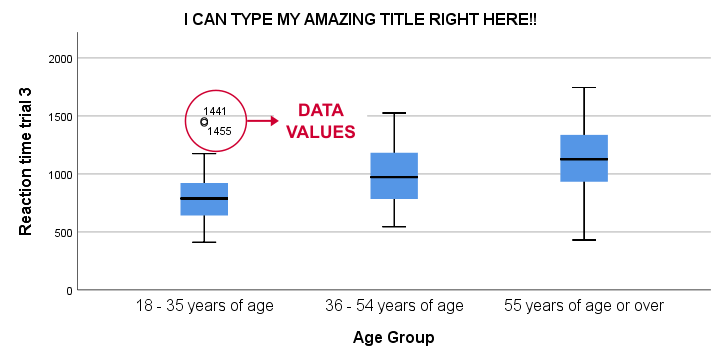This boxplot shows increasing medians and standard deviations with increasing ages. Note that our boxplot also shows outlier values. In this example, these are reaction times of 1,441 and 1,455 milliseconds but for the youngest age group only.

## Tip 1 - Remove Outliers for Single Group

If you'd like to remove outliers based on boxplot results, you'd normally set them as user missing values. For example, MISSING VALUES r03 (1441 THRU HI). sets values of 1441 and higher as missing for r03. In our example, however, this won't work: the aforementioned values are potential outliers only for the youngest age group. For the other age groups, they're within a normal range.

A solution is converting these values into different values for the youngest age group only. One option is combining DO IF with RECODE. The syntax below, however, shows a shorter option based on IF.

*Quick checktable.

means r03 by agegroup
/cells count min max mean stddev.

*Recode potential outliers into 999999998 but only for agegroup 1.

if(agegroup = 1 and r03 >= 1441) r03 = 999999998.

*Set recoded outliers as user missing values.

missing values r03 (999999998).

*Apply value label to recoded outliers.

add value labels r03 999999998 'Value removed because outlier'.

*Rerun checktable.

means r03 by agegroup
/cells count min max mean stddev.

## Tip 2 - Show Outlier Values in Boxplot

You can show data values for potential outliers and extreme values in boxplots. This only works if each boxplot involves a single dependent variable. Simply use this dependent variable as the ID variable too.
The only dialog that supports this is the Chart Builder. If you prefer the other dialogs, modifying the /ID subcommand in the syntax also does the trick.

*Show potential outlier values and extreme values -if any- in boxplot.

EXAMINE VARIABLES=r03 BY agegroup
/PLOT=BOXPLOT
/STATISTICS=NONE
/NOTOTAL
/ID=r03. /*Label outliers with actual data values.

## Tip 3 - Adding Titles to Boxplots

There's 3 options for showing titles in SPSS boxplots:

• create your boxplot via the Chart Builder as in example 3;
• use a chart template that has a fixed title and/or subtitle;

For this last option, open a Chart Editor window by double-clicking your chart. You can now add a title from the Options menu.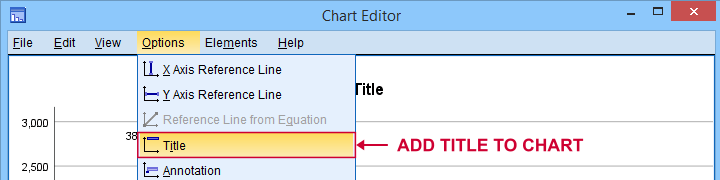## Final Notes

There's many more variations on boxplots, especially clustered boxplots. However, I think you'll get them done fairly easily after studying this tutorial.

If you've any questions or remarks, please throw me a comment below.

# Tell us what you think!

*Required field. Your comment will show up after approval from a moderator.

# THIS TUTORIAL HAS 9 COMMENTS:

•### By Jon K Peck on January 20th, 2021

There is another place to get boxplots in Statistics. The STATS REGRESS PLOT extension command is designed to screen possible predictors for regression. It produces a variety of plots based on the predictor measurement level, and for categorical variables, one of the choices is boxplots.

•### By Ruben Geert van den Berg on January 21st, 2021

Hi Jon!

I'm not a fan of boxplots in the first place and especially not for ordinal variables.

I feel the quartiles are too coarse for highly discrete variables: oftentimes, the median is equal to quartile 1 or 3. Also, subsamples and/or variables often have similar medians/quartiles, especially if there's on 4 or 5 answer categories.

On top of that, the IQR and potential outliers are based on numeric calculations (subtraction, multiplication) which are controversial for anything but quantitative variables.

But anyway, I remember the STATS_REGRESS_PLOT extension doing some nice stuff indeed. I'll look into it once more some time soon.

•### By Scott Zimmerman on February 23rd, 2022

This site is absolute gold! I am taking an online statistics course where clear instruction is difficult because of the format and because this is my first real exposure to statistics. This is my go-to site and I really appreciate what you've done here!

•### By DJINEKOU on May 2nd, 2022

Très pratique

•### By Jon Peck on May 2nd, 2022

Boxplots are a great chart type. I want to point out two places to use them in the context of regressions.

In looking at possible regression specifications graphically, you can use the STATS REGRESS PLOTS extension command (Graphs > Regression Variable Plots), available via the Extensions > Extension Hub menu if not already installed, to get a compact representation of the bivariate relationships. One of the options is to show boxplots for categorical variables. Try this syntax, which was generated from the dialog, on the dataset used here.
STATS REGRESS PLOT YVARS=r01 r02 r03 r04 r05 XVARS=sex agegroup
/OPTIONS CATEGORICAL=BOXPLOT GROUP=5 BOXPLOTS INDENT=15 YSCALE=75
/FITLINES APPLYTO=TOTAL.

Another nice place to use boxplots is in inspecting regression residuals for functional form problems and heteroscedasticity. Using the STATS RESIDUAL BOXPLOTS extension command, you can quickly spot these problems. This example uses the employee data.sav file shipped with Statistics. First you run the regression saving the predicted values and residuals.
REGRESSION
/DEPENDENT salary /METHOD=ENTER educ jobtime
/SAVE ZPRED SDRESID.
and then this syntax, which was generated from the Graphs > Regression Residual Plots menu.
STATS RESIDUAL BOXPLOTS DEPENDENT=salary
PREDVALS=ZPR_1 RESIDUALS=SDR_1 LOESS=YES.